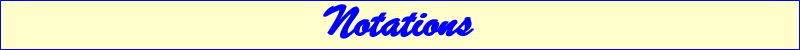The notations employed for cubics are the following : K (read $\mathcal{K}$) : a general cubic (circum-scribed or not). K+ : a cubic with three (real) concurring asymptotes. K++ : a K+ with asymptotes concurring on the curve. K60 : a cubic with three real asymptotes making 60° angles with one another. (see Special Isocubics §§5, 6 ,7 in Downloads page.) K60+ : a K60 with three (real) concurring asymptotes i.e. a stelloid. See CL006 K60++ : a K60+ with asymptotes concurring on the curve i.e. a central stelloid pK : a pivotal isocubic (see Special Isocubics §1.4 in Downloads page.) pK(W, P) : pK with pole W, pivot P. pK(M<->N, P) or pK(M x N, P) : pK with pivot P, invariant in the isoconjugation which swaps M and N. nK : a non-pivotal isocubic (see Special Isocubics §1.5 in Downloads page.) nK0 : a nK without term in xyz. nK0(W, P) : nK0 with pole W, root P. nK0(M<->N, P) or nK0(M x N, P) : nK0 with root P, invariant in the isoconjugation which swaps M and N. nK(W, P, M) : the nK with pole W, root P passing through M. cK : a conico-pivotal isocubic (see Special Isocubics §8 in Downloads page.) cK(#S, P) : cK with singularity S, root P (see Special Isocubics §8 in Downloads page.) iK : a self-inverse isocubic in the circumcircle (see Special Isocubics §4.4 in Downloads page.) All these notations combine easily e.g. pK+, pK60, nK60++, etc. A1(P), A2(P) : a generalized Allardice cubic. K(P) : a generalized Lemoine cubic. See bibliography. O(P) : an orthopivotal cubic. See bibliography. T(P) : a generalized Tucker cubic. See Downloads page. C(k), D(k) : cubics found in "Two Remarkable Pencils..." see Downloads page. Z(P), Z(U, P), etc : cubics defined by Clark Kimberling. See TCCT p. 240 and "Cubics associated with triangles of equal areas", FG vol.1, 2001. Z(U,P) = pK(W,U) where W is the barycentric product of I = X(1) and the isogonal conjugate of P. Z(P) = Z(P,I) = pK(X6,P). psK : pseudo-pivotal cubics. See here. spK : see CL055. SpK : see CL056. *** Other notations : X(i) : a center in Clark Kimberling's ETC E(i) : a center in Edward Brisse's catalogue of centers Usual triangle centers : I : incenter X(1), G : centroid X(2), O : circumcenter X(3) H : orthocenter X(4), N : nine-point center X(5) K : Lemoine point X(6), L : de Longchamps point X(20). J. H. Conway's notations : SA (read $S_A$) = (b^2 + c^2 - a^2)/2, SB, SC similarly SO = SΩ = (a^2 + b^2 + c^2)/2 sa (read $s_a$) = (b + c - a)/2, sb, sc similarly so = (a + b + c)/2